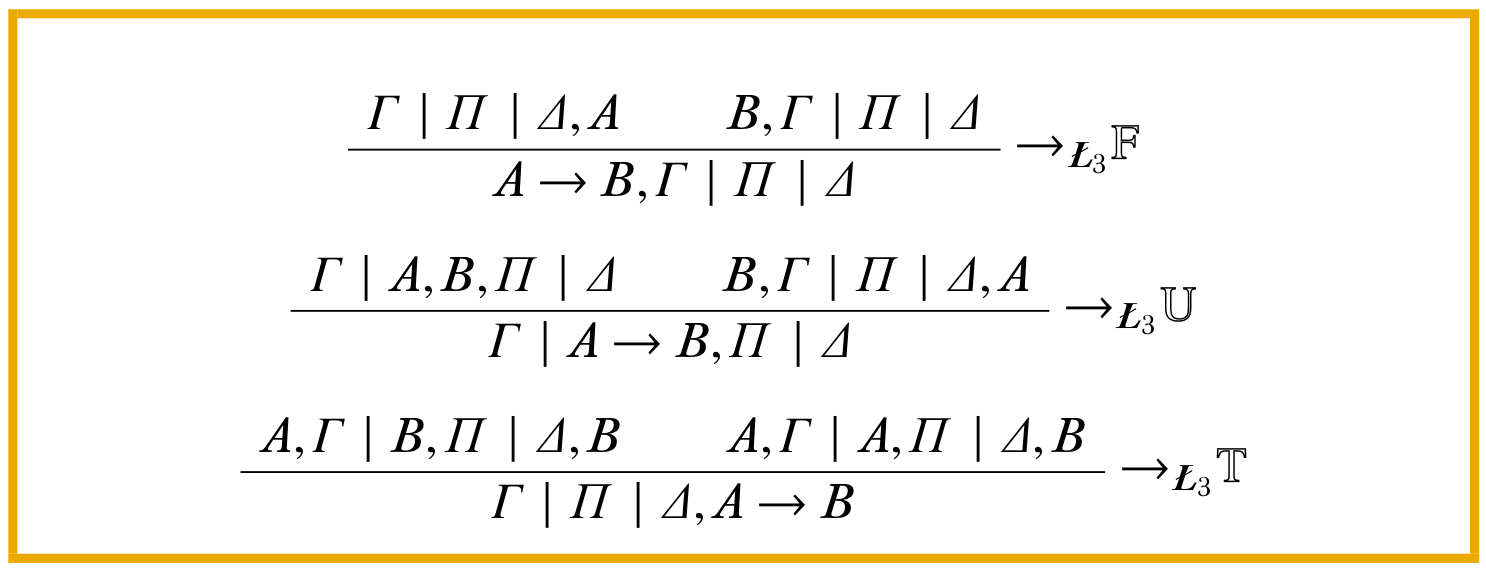Many-valued logic in the OLP

We have four new chapters in the OLP. They contain draft material on many-valued logics. The Introduction explains their syntax and semantics in general terms. A chapter on three-valued logics discusses some examples: Łukasiewicz, strong and weak Kleene, and Gödel logics (all three-valued) and the logic of Halldén, LP, and RM3. The third chapter discusses infinite-valued Łukasiewicz and Gödel logics. A fourth chapter describes n-sided sequent calculi and gives rules for the logics discussed in the three-valued logic chapter.

The chapter is part of the complete PDFs, and available separately at:

http://builds.openlogicproject.org/content/many-valued-logic/many-valued-logic.pdf